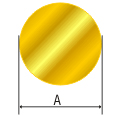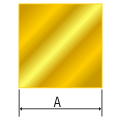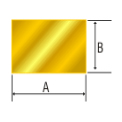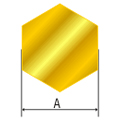## Weight Calculator

 Round RodsA mm In Brass In Copper Kg Kg Weight per Meter = (A x A x 0.006676) Kg
 Square RodsA mm In Brass In Copper Kg Kg Weight per Meter = (A x A x 0.008500) Kg
 Rectangle Rods / StripsA B mm mm In Brass In Copper Kg Kg Weight per Meter = (A x B x 0.008500) Kg
 Hex RodsA mm In Brass In Copper Kg Kg Weight per Meter = (A x A x 0.007361) Kg

A & B are in mm

### Weight of Copper Rods = 1.0517 x The Corresponding Weight of Brass Rods

1 Meter = 100 CMS = 1000 MM = 39.37 INCHES = 3.28 FEET